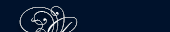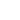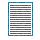НОВОСТИ   БИБЛИОТЕКА   ЭНЦИКЛОПЕДИЯ   КАРТА САЙТА   ССЫЛКИ   О САЙТЕ## SURFACES

Solids are bounded by surfaces. These surfaces separate the solids from the surrounding space. Surfaces are of two kinds: plane and curved. The surfaces of a cube, rectangular prism, pyramid are plane surfaces, while the surface of a sphere is curved. The nature of a surface may be tested as follows: place a straight edge on the surface in several positions,, If the straight edge is in contact with the surface throughout its whole length in all positions, then the surface is plane and is referred to as a plane surface or simply as a plane. If the surface is not in contact with the straight edge in all positions, then the surface is a curved surface. A sheet of paper, e. g. a leaf of a book, may represent a surface, but even the thinnest sheet of paper will be a geometrical solid, since it has length, breadth, and thickness. Each leaf of a book is a geometrical solid, the words on the pages are two of its bounding surface.ПОИСК: